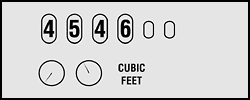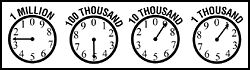There are two types of gas meters, digital and dial. Each type has distinct reading rules.

Digital Gas MetersDigital meters are easy to read. Simply read the meter and submit the numbers you see. Here's an example of a digital meter:Dial Gas MetersDial gas meters are a bit trickier to read. They turn like clocks, but in opposite directions (as does your electric meter). Each dial has the digits 0 thru 9. Each number is 1/10th (one-tenth) of a complete turn. When it makes one complete turn, the digit to its left advances to its next number.... (Slowly, like on a clock). When a dial hand is between two digits, you read the LOWER digit. (Exception to the rule: Nine and Zero. ZERO counts as TEN-TENTHS, or ONE COMPLETE TURN, so nine is the lower number.) The numbers work just like a car's odometer... after you go 9/10th (nine-tenths) of a mile, the digit to the left advances by one.

Our meter readers read meters from RIGHT to LEFT. Thinking in terms of tenths, each dial represents how many tenths the dial to the left has passed the lower of the numbers it is near. For instance, on this reading:

The dial on the right is pointing near the 1. This indicates that the dial to its left is almost 1/10th (one-tenth) past the nine. Likewise, the nine means "nine-tenths past the four". (Note: It is not saying 5 because the dial to its right has not reached the zero yet!) Therefore, this reading would be 2490.Why? Starting reading from the right: 0, that's zero-tenths past 9, nine-tenths past 4, four-tenths past 2.